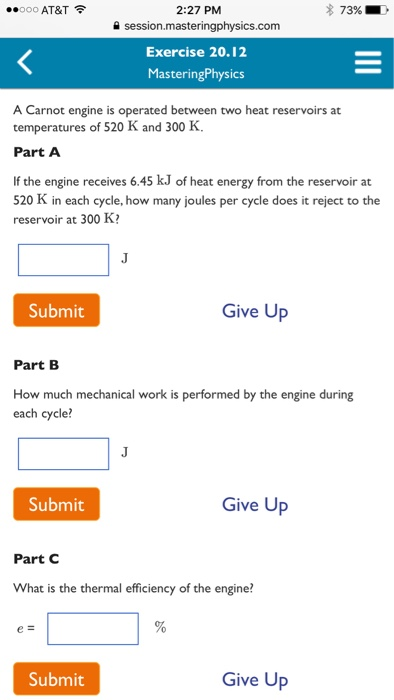# A carnot engine is operated between two heat reservoirs at temperatures of 520 k and 300 k.A Carnot engine is operated between two heat reservoirs at temperatures of 520 K and 300 K. If the engine receives 6.45 kJ of heat energy from the reservoir at 520 K in each cycle, how many joules per cycle does it reject to the reservoir at 300 K? How much mechanical work is performed by the engine during each cycle? What is the thermal efficiency of the engine?

Part A
For ideal Carnot, engine entropy is not produced in the engine.
So the sum of entropy transfer to the engine equals zero
now Q-hot/T-hot + Q-cold/T-cold = 0
Q-cold =- (Q-hot /T-hot ) * T-cold
= – 6.45kJ * 300K / 520K = – 3.72kJ
here negative sign indicates, that heat is transferred from the
engine
Part B .
W + Q-hot + Q-cold = 0
so, – W = Q-hot + Q-cold
= 6.45kJ – 3.72kJ
= 2.73kJ
Part C ..
e = -(W/Q-hot) = 2.73kJ /6.45kJ
= 0.423
= 42.3%